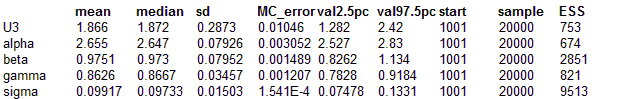#### Dugongs: nonlinear growth curve

Carlin and Gelfand (1991) present a nonconjugate Bayesian analysis of the following data set from Ratkowsky (1983):The data are length and age measurements for 27 captured dugongs (sea cows). Carlin and Gelfand (1991) model this data using a nonlinear growth curve with no inflection point and an asymptote as Xi tends to infinity:

Yi ~ Normal(μi, τ),   i = 1,...,27

μi = α - βγXi   α, β > 0; 0 < γ < 1

Standard noninformative priors are adopted for α, β and τ, and a uniform prior on (0,1) is assumed for γ. However, this specification leads to a non conjugate full conditional distribution for γ which is also non log-concave. The graph and corresponding BUGS code is given below

model
{
for( i in 1 : N ) {
Y[i] ~ dnorm(mu[i], tau)
mu[i] <- alpha - beta * pow(gamma,x[i])
}
alpha ~ dunif(0, 100)
beta ~ dunif(0, 100)
gamma ~ dunif(0.5, 1.0)
tau ~ dgamma(0.001, 0.001)
sigma <- 1 / sqrt(tau)
U3 <- logit(gamma)
}

##### Data
```    list(x = c( 1.0, 1.5, 1.5, 1.5, 2.5, 4.0, 5.0, 5.0, 7.0,   8.0, 8.5, 9.0, 9.5, 9.5, 10.0, 12.0, 12.0, 13.0,   13.0, 14.5, 15.5, 15.5, 16.5, 17.0, 22.5, 29.0, 31.5),   Y = c(1.80, 1.85, 1.87, 1.77, 2.02, 2.27, 2.15, 2.26, 2.47,   2.19, 2.26, 2.40, 2.39, 2.41, 2.50, 2.32, 2.32, 2.43,   2.47, 2.56, 2.65, 2.47, 2.64, 2.56, 2.70, 2.72, 2.57), N = 27) ```
##### Inits for chain 1
``` list(alpha = 1, beta = 1, tau = 1, gamma = 0.9) ```

##### Inits for chain 2
``` list(alpha = 0.1, beta = 0.1, tau = 0.1, gamma = 0.6) ```

Resultsmodel
{
for( i in 1 : N ) {
Y[i] ~ dnorm(mu[i], tau)
mu[i] <- alpha - beta * pow(gamma,x[i])
diffMuAlpha[i] <- diff(mu[i], alpha)
diffMuBeta[i] <- diff(mu[i], beta)
diffMuGamma[i] <- diff(mu[i], gamma)
pow[i] <- pow(gamma,x[i])
}
alpha ~ dunif(0, 100)
beta ~ dunif(0, 100)
gamma ~ dunif(0.5, 1.0)
tau ~ dgamma(0.001, 0.001)
sigma <- 1 / sqrt(tau)
U3 <- logit(gamma)
}